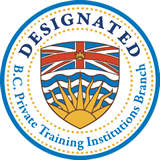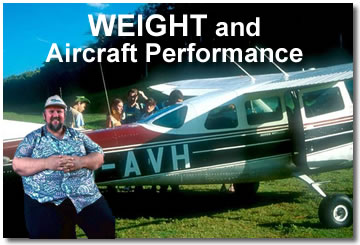HOME PAGE FLIGHT TRAINING STUDENT RESOURCES ONLINE GROUND SCHOOL CHARTER SERVICE SCENIC FLIGHTS OUR AIRCRAFT PILOT SUPPLIES AERIAL PHOTOGRAPHY CFI INSIGHTS WHY CHOOSE PRINCIPAL AIR PHOTO GALLERY AVIATION LINKS CONTACT US
 Phone: 604-850-0290 Email: info@principalair.ca Unit D 30460 Liberator Ave. (Just past the Main Terminal)Abbotsford International AirportV2T 6H5 follow us on:“Gravity never loses. The best you can hope for is a draw.”

--Aviation 101--

Preparing for a flight? Completion of a weight and balance calculation is an essential part of the process.

For commercial flights and for other “formal” occasions, for example your flight test or check ride, the weight and balance procedure will be written out and detailed. For casual, recreational flights, the process may be a bit less formal, but we are still legally responsible for ensuring the aircraft is within its limits, both in terms of overall weight and C of G position.

The weight of an aircraft is important for the whole duration of a flight, not just at take-off. Many aircraft, particularly aircraft with complex fuel systems, are capable of both changing their weight as a result of fuel burn and moving their C of G.

Many aircraft are able to take off at a weight well in excess of their maximum landing weight. The newer C-182’s, as one example, have a maximum take-off weight of 3100 lbs. The maximum landing weight is 2950 lbs.

Leaving the C of G problem for another day, let’s take a look at weight. Weight is a factor in all aspects of aircraft performance. Stall speed, maximum range airspeed, maximum endurance airspeed, maximum distance and minimum sink glide speeds, rate of climb and manoeuvring speed are all examples of aircraft performance tied directly to aircraft weight.

Back in ground school we learned about the four forces involved in aircraft flight: lift, weight, thrust and drag. Thrust opposes drag. Lift opposes weight. We say that these forces act as couples.

If opposing forces are equal, for example if thrust equals drag and lift equals weight, we say the aircraft is in a state of equilibrium; aircraft performance will remain constant. However, if lift exceeds weight, the opposing forces of lift and weight are no longer equal. The aircraft is no longer in equilibrium and a change in flight performance will occur. In this case, when lift exceeds weight, the aircraft will accelerate vertically.

If we can put some numbers to formulas for a couple of specific examples, without getting lost in the mathematics, it makes it very easy to see the relationship between aircraft weight and performance. Let’s take a look at the stall and at rate of climb. First, the stall.

The formula for lift, which we all remember from ground school, is: Lift = CLS(r/2)V2. The formula tells us that lift is equal to one-half the product of four factors:

· The Angle of Attack. Increasing the angle of attack increases the amount of lift and reaches its maximum value at the Critical Angle of Attack. Increasing the angle of attack beyond the critical angle of attack results in a significant reduction of lift: a stall. The point at which the stall is reached depends on the shape of the wing profile. This is expressed in the formula for lift by the Coefficient of Lift (CL); the critical angle of attack, the angle at which maximum lift is produced, is represented as CLmax.
· The Square of the Airspeed (V2). Increasing airspeed results in a significant increase in lift. We can see from the formula that lift increases with the Square of the Velocity. If we double the airspeed, we increase lift four fold.
· Air Density (r). Density decreases with altitude and increased temperature. Lower density results in less lift.
· Wing Surface (S). Larger wing surfaces produce more lift.

For our purposes, we’ll say that CL, S and r remain constant. Once our aircraft is in flight at a given altitude we can make the assumption that the surface area of our wings, baring some tragic occurrence, and the air density will remain constant. Since we are speaking of the stall, which occurs at a fixed angle of attack, we can consider CL as a constant using the value for CLmax.

To simplify our lives as we look at the issue of weight, we can assign the product of the factors for CL, S and r in the formula for lift a constant value, let’s say “K”. So we can look at our formula, in its simplified form, as Lift = KV2: lift is equal to K times the square of the velocity.

Let’s use a typical training aircraft which most of us have flown at one point or another for numbers: the C-172. According to the POH for the 1976 M model, the stall speed, depending on the position of the centre of gravity, is approximately 50 KCAS at gross weight, 2300 lbs. This gives us some numbers to work with.

Reminding ourselves that in un-accelerated flight lift is equal to weight, we can say that weight is also equal to our constant “K” times the square of the velocity (W = KV2).

If we plug in our numbers and solve for K, we can apply that number using weights other than full gross. V = 50 KCAS (from the POH); W = 2300 lbs. Using the formula we see that 2300 = K x 2500 or we could express this as K = 2300/2500. Solving for K we find, in this case, K = 0.92.

Let’s see what happens to the stall speed when we reduce the weight to, say, 1800 lbs., a reduction of 500 lbs., 22% of our gross weight. Now, V2 =1800/0.92. In this case we find our 1g stall speed, at 1800 lbs., would be 44.2 KCAS, a 12% reduction in the stall speed.

A rule of thumb suggested by William Kershner (1) for finding the effects of weight change on airspeed is to, “…reduce the airspeed by one-half of the percentage of the Weight reduction”. In this case that would give us a “rule of thumb” stall speed of approximately 44.5 KCAS. It isn’t quite as precise, perhaps, but it certainly is in the ballpark.

I don’t know about your eyes, but I would certainly have a difficult time finding the difference on the airspeed indicator between 44.2 and 44.5.

Rate of climb is another performance factor dependent on the flying weight of the aircraft. Rate of climb is a function of two factors: excess thrust horsepower and weight. It is generally expressed by the formula: Rate of Climb is equal to Excess Thrust Horse Power times 33,000 divided by Weight (R/C=ETHP x 33,000/W) (2).

33,000 (550 x 60) is simply the conversion of horsepower, which is normally expressed in foot-pounds per second, into foot-pounds per minute (3). We normally measure rate of climb in terms of feet per minute. It reduces confusion to keep our units consistent. I don’t know about you, but I certainly have a sufficiency of confusion in my life, already.

Going back to our C-172 POH, we find, under standard conditions at gross weight, Cessna tells us we can expect a rate of climb of 645’/min at sea level. Plugging this information into our formula for Rate of Climb we find that the aircraft produces approximately 45 Excess Thrust Horse Power (645 @ 45 x 33,000/2300) under these conditions.

If we reduce our weight to 1800 lbs. again and run the formula, assuming the same excess thrust horse power—this is simplifying just a bit, but it demonstrates the point—we find that the Rate of Climb has increased to 825’/minute, a 128% increase (R/C = 45 x 33,000/1800).

The teacher in me wants to say here, “So, what can we learn from this?” On a practical, day to day level: increase weight, increase stall speed; decrease weight, increase rate of climb, and visa versa. If we need to get more specific, we can always do the math.

We’ve all been tempted at one time or another to have a go at a short runway possibly with an obstacle at the end when loaded just a little on the heavy side. What a perfect moment to remember: the numbers don’t lie.

All considered it’s a healthy concept to keep in mind: the flying weight of your aircraft directly affects its performance. Each time. Every time. No exceptions.

End Notes:

1. Kershner, William K., The Advanced Pilot’s Flight Manual, Sixth Edition, Iowa State University Press, Ames, Iowa, USA, 1994, pg.7.
2. ibid, pg. 90.
3. 1 Horse Power = 550 ft-lbs/second or 33,000 ft-lbs/minute